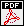# Dimers and orthogonal polynomials: connections with random matrices

Content: In these lecture notes we present some connections between random matrices, the asymmetric exclusion process, random tilings. These three apparently unrelated objects have (sometimes) a similar mathematical structure, where the correlation functions are given in terms of a kernel, which on its own is expressed in terms of orthogonal polynomials.

 • Lecture notes (updated 14. December 2009)